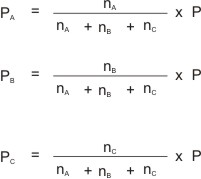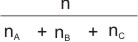## Dalton’s Law of Partial Pressure

Dalton’s law of partial pressure states that the total pressure exerted by a mixture of gases is equal to the sum of the partial pressures of the component gases, provided the gases do not react chemically with each other.

By this law, if we have a mixture of gases A, B and C, of partial pressures PA, PB and PC respectively in a vessel without them reacting, then the total pressure they exert is the sum of their partial pressures.

I.e. P = PA + PB + PC

Note: the law implies that each gas in the mixture, for as long as there is no chemical reactions taking place, exert a pressure same as when it is alone in the vessel. This pressure is known as its partial pressure.

A mixture of gases, A, B, and C, containing nA, nB and nC number of moles respectively, and exerting a total pressure of P will have their individual partial pressures derived by using their mole ratios (or mole fractions). I.e.Where PA = partial pressure of A ; PB = partial pressure of B ; PC = partial pressure of C . P = total pressure; andis the mole ratio or mole fraction of any of the gases in the mixture.

### Calculation Based on Dalton's Law of Partial Pressure

2 moles of oxygen gas, 3 moles of nitrogen gas and 1 mole of carbon(IV) oxide gas are put in a gas balloon whose pressure is maintained at 3.019 x 105 N M-2 at 28 oC. What is the partial pressure of nitrogen?

Solution:Like This Post? Please Share!!

Copyright , All Rights Reserved Free Chemistry Online | About Us | Usage of Content | Total Disclosures | Privacy Policy